# HBSE 7th Class Maths Solutions Chapter 7 Congruence of Triangles InText Questions

Haryana State Board HBSE 7th Class Maths Solutions Chapter 7 Congruence of Triangles InText Questions and Answers.

## Haryana Board 7th Class Maths Solutions Chapter 7 Congruence of Triangles InText Questions

Discuss, Think & Write (Page No. 137):

Question 1.
When two triangles, say ABC and PQR are given, there are, in all, six possible matchings or correspondences. Two of them are:
(i) ABC ⇔PQR and
(ii) ABC ⇔ QRP
Find the other four correspondences by using two cut outs of triangles. Will all these correspondences lead to congruence ? Think about it.
Solution:
For better understanding of the correspondence let us use a diagram.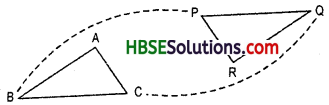(i) The correspondence is ABC ⇔ PQR. This means A ⇔ P ; B ⇔ Q ; and C ⇔ R.
So; (i) $$\overline{\mathrm{QR}} \leftrightarrow \overline{\mathrm{BC}}$$ (ii) ∠R ↔ ∠C and
(iii) $$\overline{\mathrm{PQ}} \leftrightarrow \overline{\mathrm{AB}}$$
Similarly we find the all possible matchings or correspondences, e.g.

(ii) ABC ⇔ QRP : ∠A ⇔ ∠Q, ∠B ⇔ ∠R,
∠C ⇔ ∠P
$$\overline{\mathrm{AB}} \leftrightarrow \overline{\mathrm{QR}}, \overline{\mathrm{BC}} \leftrightarrow \overline{\mathrm{RP}}, \overline{\mathrm{AC}} \leftrightarrow \overline{\mathrm{QR}}$$

(iii) ABC ⇔ XYZ,
(iv) ABC ⇔ YZX
(v) ABC ⇔ ZXY,
(vi) ABC ⇔ DEF.Try These (Page No. 140-141):

Question 1.
In Fig. lengths of the sides of the triangles are indicated. By applying the SSS congruence rule, state which pairs of triangles are congruent. In case of congruent triangles, write the result in symbolic form: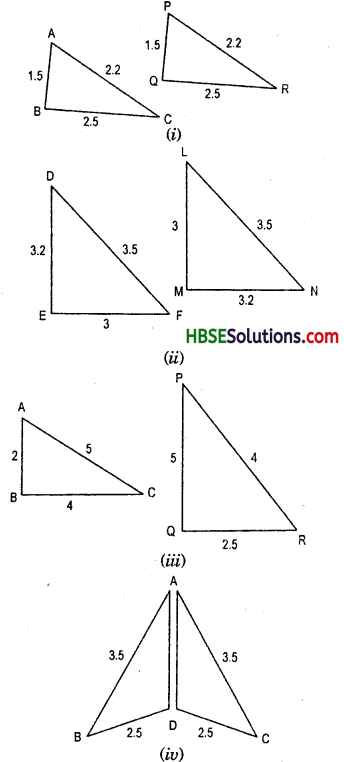Solution:
From fig(i);
In triangle ABC and PQR
AB = PQ = 1.5
BC = QR = 2.5
and AC = PR = 2.2
This shows that the three sides of one triangle are equal to the three sides of the other triangle. So, by SSS congruence rule, the two triangles are congruent. From the above three equality relations, it can be easily seen that A ⇔ P.B ⇔ Q and C ⇔ R.
So, we have ΔABC ≅ ΔPQR .
From fig. (ii);
In triangles DEF and NLM,
DE = MN = 3.2
EF = LM = 3
FD = NL = 3.5
This shows that the three sides of one triangle are equal to the three sides of the other triangle. So, by SSS congruence rule, the two triangles are congruent.
From the above three equality relations, it can be easily seen that
D ⇔ N, E ⇔ M and F ⇔ L.
So, we have ΔDEF ≅ ΔNLM.
From Fig. (iii);
In triangles ABC and PQR AB = 2, PQ = 5 ∴ AB ≠ PQ
AC = 5, PR = 4 ∴ AC ≠ PR
BC = 4, QR = 2.5 ∴ BC ≠ QR
Hence, ΔABC and ΔPQR are not congruent. From Fig. (iv);
AB = AC = 3.5
BD = DC = 2.5
This shows that the three sides of one triangle are equal to the three sides of the other triangle. So by SSS congruence rule, the two triangles are congruent.
From the above three equlity relations, it can be easily seen that
A ⇔ A,B ⇔ C and D ⇔ D.
So, we have ΔABC ≅ ΔADC.Question 2.
In Fig., AB = AC and D is the mid-point of $$\overline{\mathbf{B C}}$$
(r) State the three pairs of equal parts in ΔADB and ΔADC.
(iii) Is ∠B = ∠C Why ?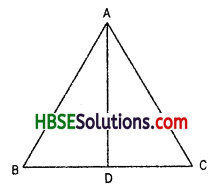Solution:
From Fig. ;
(i) AB = AC
(ii) This shows that the three sides of one triangle are equal to the three sides of the other triangle. So, by SSS congruence rule, the two triangles are congruent.
From the above three equality relations, it can be easily seen that
A ⇔ A, B ⇔ C and D ⇔ D
(iii) By corresponding parts of congruent triangles (C.P.C.T) are equals
hence, ∠B = ∠C.

Question 3.
In Fig., AC = BD and AD = BC. Which of the following statements is meaningfully written ?
(i) ΔABC ≅ ΔABD.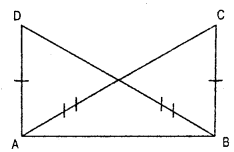Solution:
From Fig.
(i) In triangles ABC and ABD
BD = AC
AB = AB = Common
This shows that the three sides of one triangle are equal to the three sides of the other triangle. So, by SSS congruence rule, the two angles are congruent.

From the above three equality relations, it can be easily seen that
A ⇔ B, D ⇔ C and ∠CAB = ∠DBA.
So, we have AABC s AABD
(ii) Similarly, above three equality relations ∠ADB = ∠ACB, ∠ABD = ∠BAC and ∠DAB = ∠ABC.
So, we have ΔABC ≅ ΔBAD.Think, Discuss & Write (Page No. 141):

Question 1.
ABC is an isosceles triangle with AB = AC (Fig. 7.15)
Take a trace copy of ΔABC and also name it as ΔABC.
(i) State the three pairs of equal parts in ΔABC and ΔACB.
(ii) Is ΔABC ≅ ΔACB ? Why or why not ?
(iii) Is ∠B = ∠C ? Why or why not ?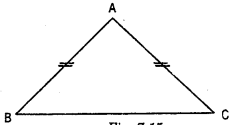Solution:
in Triangle ΔABC and ΔACB
AB = AC, AC = AB and BC = CB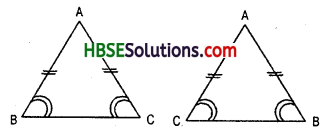But in isosceles triangle two sides are equal and base angles are equal.
Hence, ∠B = ∠C and ∠C = ∠B
From the above three equality rela-tions, it can be easily seen that, A ⇔ A, B ⇔ C and C ⇔ B
So, we have ΔABC ≅ ΔACB. (by SAS congruence rule)

(i) AB = C, AC = AB, BC = CB
(ii) Yes, ΔABC ≅ ΔACB
(iii) Yes, ∠B = ∠C. (by CPCT and by isosceles triangle).

Try These (Page No. 143-144):

Question 1.
Which angle is included between the sides DE and EF of ΔDEF ?
Solution:
∠DEF or ∠E is included between the sides DE and EF of ΔDEF.

Question 2.
By applying SAS congruence rule, you want to establish that ΔPQR s ΔFED. It is given that PQ – EF and RP = DE What additional information (pair of equal parts) is needed to establish the congruence ?
Solution:
In ΔPQR and ΔDEF,
PQ = EF =3 and RP = DF = 3.5
If ∠F = ∠P then the ΔPQR ≅ ΔFED
Since, ∠F = 40° but ∠P is not given.

Question 3.
In Fig., measures of some parts of the tirangles are indicated, by applying SAS congruence rule, state the pairs of congruent triangles, if any, in each case. In case of congruent triangles, write them in symbolic form.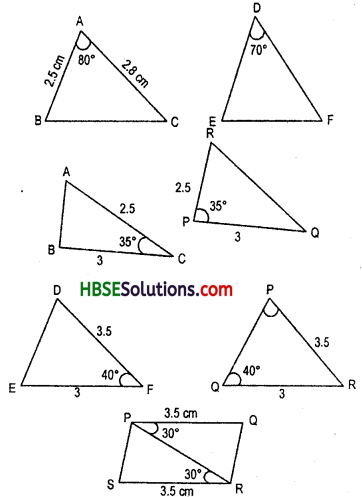Solution:
From Fig. 7.17, ΔACB ≅ ΔRPQ;
ΔPRS ≅ ΔRPQ
Now, In ΔACB and ΔRPQ
AC = RP =2.5,
BC = PQ = 3
and included ∠C = included
∠P =35°
Also, A ⇔ R , B ⇔ Q and C ⇔ P

Therefore, ΔACB = ΔRPQ (By SAS congruence rule)
Now, in a ΔPRS and ΔRPQ
PR = RP = Common
RS = PQ =3.5
and included ∠R = included ∠P = 30°
Also, R ⇔ P.S ⇔ Q and P ⇔ R
Thererefore, ΔPRS ≅ ΔRPQ (By SAS congruence rule)Question 4.
In Fig. $$\overline{\mathbf{A B}}$$ and $$\overline{\mathrm{CD}}$$ bisect each other at O
(i) State the three pairs of equal parts in two triangles AOC and BOD.
(ii) Which of the following statements are true ? (Fig.)
(a) ΔAOC ≅ ΔDOB
(b) ΔAOC ≅ ΔBOD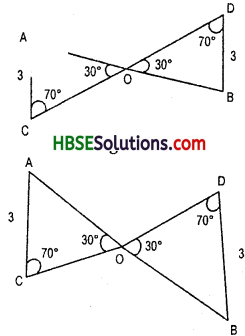Solution:
(i) From Fig.
AB and CD bisect each other at O.
Therefore, AO = OB, CO = OD,
AC = DB
and ∠AOC = ∠DOB = Vertically opposite angles
= 30°
∠ACO = ∠BDO = alternate interior angle
= 70°
Hence, ∠CAO = ∠DBO = alternate interior angle

ii) (a) In ΔAOC and ΔDOB
AC = DB = 3,
CO = OD

and included ∠C = included
∠D =70°
Also, A ⇔ B,C ⇔ D and O ⇔ O
Therefore, ΔAOC ≅ ΔDOB
(6) In AAOC s ABOD
AO = OB,
CO = OD and
Included angle ∠AOC = included angle ∠BOD = 30°
Also, A ⇔ B, C ⇔ D and O ⇔ O
Therefore, ΔAOC ≅ ΔBOD
(By SAS congruence rule)Try These (Page No. 145-146):

Question 1.
What is the side included between the angles M and N of MNP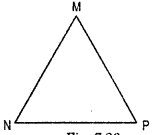Solution:
Side MN = MP
included angle = ∠NMP
and side NM = NP
included angle = ∠MNP

Question 2.
You want to establish ΔDEF = ΔMNP, using the ASA congruence rule. Your are given that ∠D = ∠M and ∠F = ∠P. What information is needed to establish the congruence ? (Draw a rough figure and then try !)
Solution:
In ΔDEF and ΔMNP,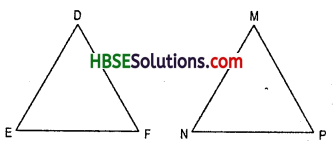∠D = ∠M, ∠F = ∠P and DF = MP i.e. D ⇔ M, F ⇔ P and DF ⇔MP
For ASA congruence rule, we need the two sides which the two angles ∠D = ∠M and ∠F = ∠P are included, so the addtional information are as follows:
DF = MP
Hence ΔDEF ≅ ΔMNP.

Question 3.
In Fig., measures of some parts are indicated. By applying ASA congruence rule, state which parts of triangles are congruent. In case of congruence, write the result in symbolic form.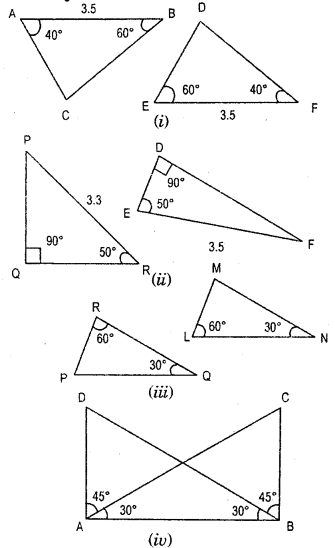Solution:
(i) In ΔABC and ΔEFD, we have
∠BAC=∠EFD = 40° (given)
∠ABD = ∠DEF = 60° (given)
AB = EF = 3.5 (given)
Hence ΔABC ≅ ΔEFD (By ASA congruence rule)

(ii) In ΔPQR and ΔDEF,
∠PQR = ∠EDF = 90° (given)
∠PRQ = ∠DEF = 50° (given)
PR = EF = 3.5 (given)
Hence ΔPQR ≅ ΔDEF (By ASA congruence rule)

(Hi) In ΔRQP and ΔLNM,
∠PRQ = ∠MLN = 60° (given)
∠PQR = ∠LMN = 30° (given)
RQ = LN = 6 (given)
Hence ΔRQP ≅ ΔLNM (By ASA congruence rule)

(iv) In ΔDAB and ΔCBA,
∠DAB = ∠CBA = (45°+ 30°)
= 75° (given)
∠ABD = ∠BAC = 30° (given)
AB = AR = common
Hence ΔDAB ≅ ΔCBA (By ASA congruence rule)

Question 4.
Given below are measurements of some parts of two triangles. Examine whether the two triangles are congruent or not, by ASA congruence rule. In case of congruence, write it in symbolic form.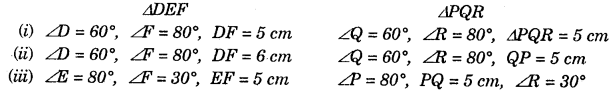Solution: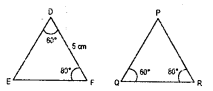If under a correspondence
By applying ASA congruence rule, ∠D = ∠Q = 60° (given)
∠F = ∠R = 80° (given)
DF = QR = 5 cm (given)

∠D = ∠Q = 60° (given)
∠F = ∠R = 80° (given)
DF = QR = 5 cm (given)
Hence ΔDEF ≅ ΔPQR(iii) In ΔDEF and ΔPQR
∠D = ∠Q = 60° (given)
∠F = ∠R = 80° (given)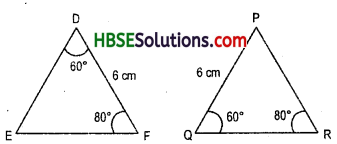But included side DF ≠ QR
Hence ASA congruence rule not satisfied.
So, ASA congruence rule cannot be applied and we cannot conclude that two triangles are congruent.

(iii) In ΔDEF and ΔPQR
∠E = ∠P = 80° (given)
∠F = ∠R = 30° (given)
But included side EF ≠ PR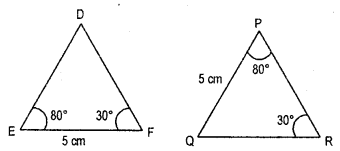So, ASA congruence rule cannot be applied and we cannot conclude that two triangles are congruent.

Question 5.
In Fig., ray AZ bisects ∠DAB as well as ∠DCB.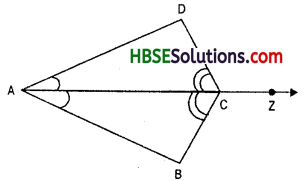(i) State the three pairs of equal parts triangles BAC and DAC.
(ii) Is ΔBAC ~ ΔDAC ? Give reasons.
(iv) Is CD = CB ? Give reasons.
Solution:
Since ray AZ bisects ∠DAB as well as∠DCB.
Hence ∠DAC = ∠BAC and ∠DCA = ∠BCA

(i) In ΔBAC and ΔDAC,
∠BAC = ∠DAC (given)
∠BCA = ∠DCA (given)
AC = AC = Common
Hence ΔBAC = ΔDAC (by ASA congruence rule)

(ii) From solution (i), by ASA congruence rule, we have
ΔBAC s ΔDAC.

(iii) Since ΔBAC = ΔDAC
hence by CPCT (corresponding part of congruent triangle)
DC = CB
and ∠D ↔ ∠B
(iv) By CPCT, CD = CB
because ΔBAC = ΔDAC.

Try These (Page No. 148):

Question 1.
In Fig., measures of some parts of triangles are given. By applying RHS congruence rule, state which pairs of triangles are congruent. In case of congruent triangles, write the result in symbolic form.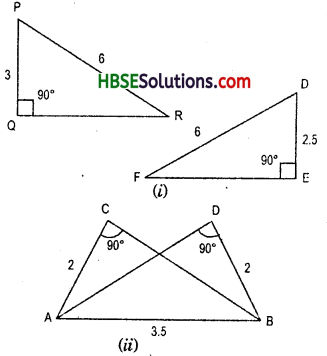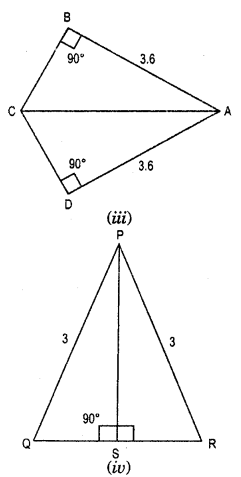Solution:
If under a correspondence the hypotenuse and one side of a right-angled triangle are respectively equal to the hypotenuse and one side of another right angled triangle, then the triangles are congruent.

(i) In ΔPQR and ΔDEF,
∠Q = ∠E = 90°
Hypotenuse PR = Hypotenuse DF = 6
Side PQ ≠ side DE
So, the triangles are not congruent.(ii) In ΔCAB and ΔDAB,
∠C = ∠D = 90°
Hypotenuse AB = Hypotenuse
AB = Common
Side CA = side DB = 2
So, ΔCAB = ΔDAB (By RHS congruence rule)

(iii) In ΔBCA and DCA,
∠B = ∠D = 90°
Hypotenuse CA = Hypotenuse
CA = Common
Side BA = side DA = 3.6
So, ΔBCA = ΔDCA (By RHS congruence rule)

(iv) In ΔPQS and ΔPRS,
∠PSQ = ∠PSR = 90°
Hypotenuse PQ = Hypotenuse PR = 3
Side PS = side PS = Common
So, ΔPQS ≅ ΔPRS.

Question 2.
Is to be established by RHS congruence rule that ΔABC S ΔRPQ. What additional information is needed, if it is given that ∠B = ∠P = 90° and AB = RP ?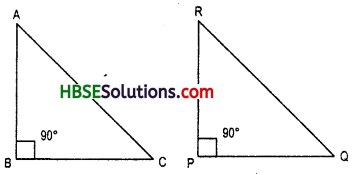Solution:
In ΔABC and ΔRPQ
∠B = ∠P = 90°
Side AB = Side RP
The additional information are as follows :
If hypotenuse AC = hypote-nuse RQ
So, ΔABC ≅ ΔRPQ.

Question 3.
In Fig. , BD and CE are altitudes of ΔABC such that BD = CE.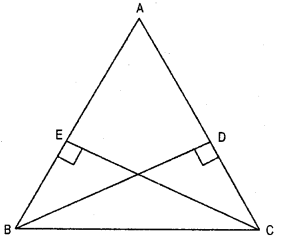(i) State the three pairs of equal parts in ΔCBD and ΔBCE.
(ii) Is ΔCBD ≅ ΔBCE ? Why or why not ?
(iii) Is ∠DCB ≅ ∠EBC ? Why or why not ?
Solution:
BD = CE,
(i) ∠BEC = ∠BDC = 90° (given)
BD = EC
BE = DC
i. e., ΔCBD = ΔBCE (SAS congruence)
(ii) Yes, because SAS congruency.
(tit) By CPCT, ∠DCB = ∠EBC. (Fig. 7.30)
hence ΔCBD ≅ ΔBCE.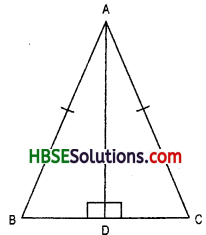Question 4.
ABC is an isosceles triangle with AB = AC and AD is one of its altitudes (Fig. 7.31)
(i) State the three pairs of equal parts in ΔADB and ΔADC.
(iii) Is ∠B = ∠C ? Why or why not ?
(iv) Is BD = CD ? Why or why not ?
Sol. Since, ABC is an isosceles triangle.
Hence, AB = AC and AD is altitude of BC.